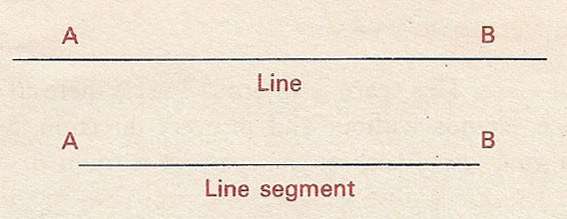A# lineFig 1. Part of a line between two points is referred to as a line segment. The line itself is infinitely long.Fig 2. The graph of y = ax + b, the prototypical linear relationship.

A line is the shortest distance between any two points in Euclidean space. A line is implicitly a straight line. It has only one dimension, length, may be infinite in extent, and is the shortest distance between any two points.

Although in common parlance a straight line is not curved, it is a curve in the mathematical sense (i.e., a mapping of an infinite set of points into a space). Mathematically, a line may be determined by the presence of any two points in an n-dimensional space (where n is two or more). A line segment is a piece of a line with definite endpoints (Fig 1).

In two dimensional Cartesian coordinates, the equation of a line has the form y = ax + b, where a and b are constants, a being the gradient of the line and b its y-intercept (Fig 2).

## Properties of straight lines

A line is uniquely determined by two points. In three points lie on a line then we can say of one of the three points that it lies between the other two. A line is divided by any one of its points into two parts. A line is unbounded in two directions.

Two straight lines which lie in one and the same plane have either one point (point of intersection) in common, or none; in the latter case they are said to be parallel. A straight line divides a plane into two parts. A line which does not lie wholly in a plane either has exactly one point in common with the plane (point of intersection, trace) or none at all; in the latter case the line is parallel to the plane.

Two straight lines that are not coplanar have no point in common. Such lines are either parallel or 'skew' with respect to each other.

## Line segment

The totality of points that lie between two fixed points A and B on a line, together with the points A and B, form a straight line segment. A and B are called the end-points of the segment (Fig 1).